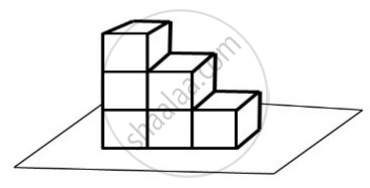# Six Dice Are Stacked on the Floor as Shown in the Figure Below. on Each Dice, the Sum of Numbers on Opposite Faces is 7, I.E., If 1 is Written on One Face Then 6 is Written on the - Mathematics

MCQ

Six dice are stacked on the floor as shown in the figure below. On each dice, the sum of numbers on opposite faces is 7, i.e., if 1 is written on one face then 6 is written on the face opposite it and so on.What is the maximum possible sum of numbers on the 21 visible faces?

• 88

• 89

• 96

• 147

#### Solution

89

Explanation:

Pair of numbers on opposite faces of the dice = (1 - 6), (2 - 5), (3 - 4)

To get maximum on visible faces, we should have the least value on invisible faces sum of the number on visible faces on top-placed dice

= 2 + 3 + 4 + 5 + 6 = 20

Sum of numbers on visible faces on middle row dice
= (6 + 5 + 2) + (6 + 4 + 4 + 3) = 31

Sum of numbers on visible faces on bottom row dice
= (6 + 5 +2) + (6 + 1) + (6 + 5 + 3 +4) = 38

Hence, total sum = 20 + 31 + 38 = 89

Concept: Probability (Entrance Exam)
Is there an error in this question or solution?